# System of different representatives

(diff) ← Older revision | Latest revision (diff) | Newer revision → (diff)

system of distinct representatives, for a given family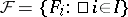of subsets of a setA set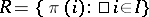determined by a one-to-one mapping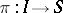that has the property:for any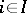(hereis an arbitrary index set). Another name for a systemof distinct representatives is transversal for the family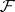. One also considers partial transversals of a family, namely sets of the form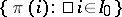, where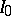is a subset ofand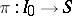is a one-to-one mapping.

Systems of distinct representatives are used both in purely combinatorial mathematical studies and in applications to linear programming, mathematical economics and cybernetics. In the framework of combinatorial mathematics, systems of distinct representatives play an important role in questions of choice and in extremal problems. They are used, in particular, in the study of Latin squares, in the assignment problem, in various extremal problems, in min-max theorems, and in the study of matrices with non-negative entries and with row and column sums in given ranges.

A criterion for the existence of a system of distinct representatives for a finite setis given by Phillip Hall's theorem: On a set, let a familyof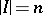elements,finite, be given; a system of distinct representatives exists if and only if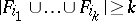for every-subset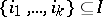and for every,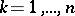. Hall's theorem is equivalent to König's theorem (see Selection theorems) about matrices of zeros and ones. This fundamental criterion can also be applied to infinite setswhen all,, are finite. Roughly speaking, as is shown by examples, such cases exhaust the domain of applicability of Hall's criterion, but it serves as a starting point for various criteria in several other cases (see ). For example: a) when there is a subset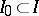for which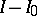is finite and the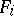are finite for all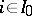; or b) whenis a countable set.

In view of the wide use of systems of distinct representatives, algorithms for their practical determination (see ) are of interest.

One of the main problems on systems of distinct representatives is the problem of the number of such systems for finite families of finite sets; this is connected with the calculation of the permanent of a matrix of zeros and ones. There are lower bounds for the number of such systems. Let the familyconsist ofsubsets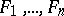and let these be ordered by cardinality: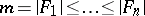. Ifsatisfies Hall's criterion, then the number of systems of distinct representatives is at least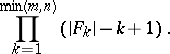Questions on systems of representatives are dealt with also in the theory of matroids (cf. Matroid, also called independence spaces or combinatorial geometries, cf. Combinatorial geometry). The connection between the theory of representatives and matroids is given by the Edmonds–Fulkerson theorem: For a given family of subsets of a finite set, the totality of all partial transversals is the totality of independent subsets of some matroid. The matroid so obtained from the familyis called the transversal matroid for. Many matroids can be represented as transversal matroids for some family of sets.

The concept of a system of distinct representatives can be generalized in various directions, for example: a)-transversals for a given familyand an integral vector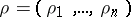are sets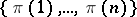, where the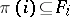,, are pairwise disjoint subsets ofsuch that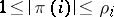; b)-transversals forand an integer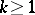are subsetsfor mappingswith the properties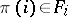and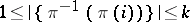,.

How to Cite This Entry:
System of different representatives. Encyclopedia of Mathematics. URL: http://encyclopediaofmath.org/index.php?title=System_of_different_representatives&oldid=15553
This article was adapted from an original article by V.E. Tarakanov (originator), which appeared in Encyclopedia of Mathematics - ISBN 1402006098. See original article